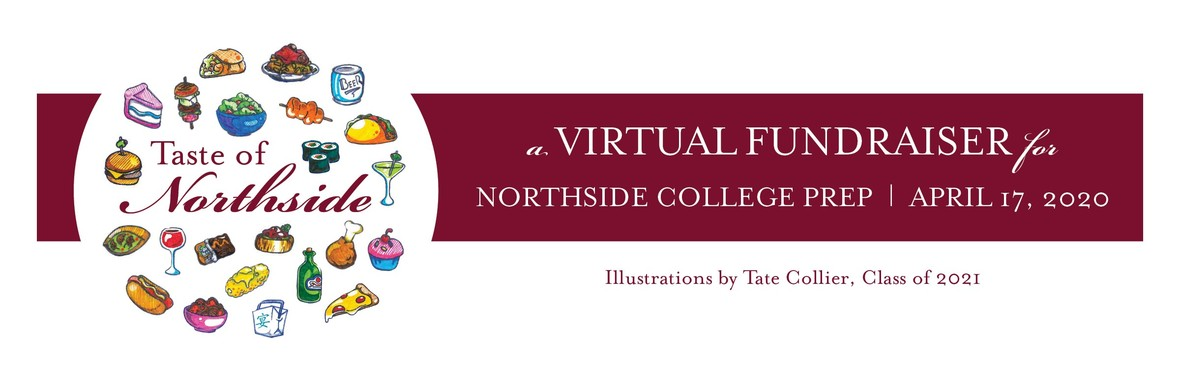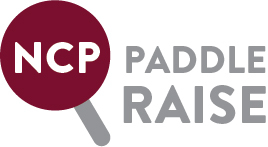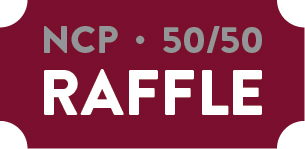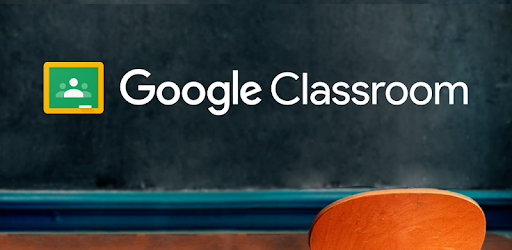/* styles */ Everyone is a “Friend of Northside” and we know these are unique and trying times for our community. We understand communication is key, so the FON Board is working hard to keep you updated on what’s happening in the Northside community. Northside’s admin team will communicate weekly academic news while this Sunday eBlast will focus on ways to support and learn more about NCP. Please reach out to us at thisweek@friendsofnorthside.org with any news we can share with the greater NCP community. We are stronger when we work together to keep connected. Go Mustangs and be well!
 table div table+table+table div table{width:100%;padding:0}table div table+table+table div table img{width:96.23%;padding:0;float:none}table div table+table+table div table td{width:100%;padding:0 1.88% 18px}/* styles */## Taste of Northside Virtual Event

 /* styles */ Friday, April 17 | 7:00 - 9:00 pm Although the in-person event was cancelled, the Northside community will be able to participate in the Live Auction, Paddle Raise, and Raffle announcement during our virtual event on Friday, April 17 from 7:00 - 9:00 pm. Be sure to register to participate.

## Premier Auction

 /* styles */ Our fantastic live auction items will be available for bidding. You can preview them HERE.
 table div table+table+table+table+table+table+table+table+table+table div table td,table.module-9{width:100%;padding:0}table div table+table+table+table+table+table+table+table+table+table div table{width:100%;float:none;margin-left:auto;margin-right:auto;padding:0}table div table+table+table+table+table+table+table+table+table+table div table a{border:0 none;text-decoration:none}table div table+table+table+table+table+table+table+table+table+table div table img{width:100%!important;border:0 none;text-decoration:none}/* styles */
 table div table+table+table+table+table+table+table+table+table+table+table div table td,table.module-10{width:100%;padding:0}table div table+table+table+table+table+table+table+table+table+table+table div table{width:100%;float:none;margin-left:auto;margin-right:auto;padding:0}table div table+table+table+table+table+table+table+table+table+table+table div table a{border:0 none;text-decoration:none}table div table+table+table+table+table+table+table+table+table+table+table div table img{width:100%!important;border:0 none;text-decoration:none}/* styles */

## Northside Needs a New FieldThis year's Paddle Raise will raise funds for a much-needed, new turf athletic field. Every year, more gym classes and home athletic games must be cancelled because our Northside field is in such poor condition. Heavy use over the years has rendered it virtually unusable. A new turf field will allow our students, and future Northsiders, to play and exercise outside safely throughout the year. Let's give all of them a home-field advantage. Please demonstrate your strong support for this long-overdue improvement to the Northside campus by making a monetary contribution to the 2020 Paddle Raise.

You don't have to wait until April 17 to participate in the Paddle Raise for NCP's new turf field. Our Northside video club, the Mustang Reel, created THIS VIDEO to inspire your generosity. Let's all PONY UP, and then click HERE to see the impact of your contribution.

## Buy Raffle Tickets for a Chance to Win!Help us reach this year's goal of \$10,000 in raffle sales! The drawing is on April 17. Click HERE to buy one chance for \$25 and HERE to purchase five chances for \$100.

# Silent Auction Item Pick-Up

Congratulations to everyone who won items during our great silent auction!

To pay any remaining open balance, please go to Northside.GiveSmart.com.

 /* styles */ Questions? Email Debra Swan, Event Chair at debra@theswans.net.
 table div table+table+table+table+table+table+table+table+table+table+table+table+table+table+table+table+table+table+table+table+table div table{width:100%;padding:0}table div table+table+table+table+table+table+table+table+table+table+table+table+table+table+table+table+table+table+table+table+table div table img{width:96.23%;padding:0;float:none}table div table+table+table+table+table+table+table+table+table+table+table+table+table+table+table+table+table+table+table+table+table div table td{width:100%;padding:0 1.88% 18px}/* styles *//* styles */ Follow Northside on Instagram: https://www.instagram.com/northsidecollegeprep/ Follow Northside's Asian club on Instagram: https://www.instagram.com/ncpaasa/
 table div table+table+table+table+table+table+table+table+table+table+table+table+table+table+table+table+table+table+table+table+table+table+table+table+table div table{width:100%;padding:0}table div table+table+table+table+table+table+table+table+table+table+table+table+table+table+table+table+table+table+table+table+table+table+table+table+table div table img{width:96.23%;padding:0;float:none}table div table+table+table+table+table+table+table+table+table+table+table+table+table+table+table+table+table+table+table+table+table+table+table+table+table div table td{width:100%;padding:0 1.88% 18px}/* styles */Remote Learning begins Monday, April 13.

Read the NCP Communication from Saturday, April 04 HERE.

Read the NCP Communication from Monday, March 30 HERE.

 table div table+table+table+table+table+table+table+table+table+table+table+table+table+table+table+table+table+table+table+table+table+table+table+table+table+table+table+table div table{width:100%;padding:0}table div table+table+table+table+table+table+table+table+table+table+table+table+table+table+table+table+table+table+table+table+table+table+table+table+table+table+table+table div table img{width:96.23%;padding:0;float:none}table div table+table+table+table+table+table+table+table+table+table+table+table+table+table+table+table+table+table+table+table+table+table+table+table+table+table+table+table div table td{width:100%;padding:0 1.88% 18px}/* styles */Parents can gain insight into what is required for each class directly from your student’s teacher. Ask your student for contact info for each teacher and send an email requesting a weekly Google Classroom summary. Student engagement is one way teachers are making sure students are “back to learning” and the summaries are a good way to start a dialogue with your student.

 table div table+table+table+table+table+table+table+table+table+table+table+table+table+table+table+table+table+table+table+table+table+table+table+table+table+table+table+table+table+table+table div table{width:100%;padding:0}table div table+table+table+table+table+table+table+table+table+table+table+table+table+table+table+table+table+table+table+table+table+table+table+table+table+table+table+table+table+table+table div table img{width:96.23%;padding:0;float:none}table div table+table+table+table+table+table+table+table+table+table+table+table+table+table+table+table+table+table+table+table+table+table+table+table+table+table+table+table+table+table+table div table td{width:100%;padding:0 1.88% 18px}/* styles */# Parent Questions - For Parents Only

As a reminder, this Question Form is available to submit your questions to the NCP administration. Please note that you will not receive a direct response to your question. Instead, this form will be used as a repository for parent questions. Once the admin team receives information or finds answers to these questions, they will be sent out as part of the parent updates.

 table div table+table+table+table+table+table+table+table+table+table+table+table+table+table+table+table+table+table+table+table+table+table+table+table+table+table+table+table+table+table+table+table+table div table{width:100%;padding:0}table div table+table+table+table+table+table+table+table+table+table+table+table+table+table+table+table+table+table+table+table+table+table+table+table+table+table+table+table+table+table+table+table+table div table img{width:96.23%;padding:0;float:none}table div table+table+table+table+table+table+table+table+table+table+table+table+table+table+table+table+table+table+table+table+table+table+table+table+table+table+table+table+table+table+table+table+table div table td{width:100%;padding:0 1.88% 18px}/* styles *//* styles */ Dear Parents & Friends of the Class of 2020, To commemorate your student's accomplishments or declare your best wishes, The Stampede, Northside's yearbook, offers ad space in its upcoming edition. The prices are as follows: full page \$150.00; half page \$80; quarter page \$50. Please see the order form for details: All materials are due by Monday, April 13th. Thank you for your interest!
 table div table+table+table+table+table+table+table+table+table+table+table+table+table+table+table+table+table+table+table+table+table+table+table+table+table+table+table+table+table+table+table+table+table+table+table+table div table{width:100%;padding:0}table div table+table+table+table+table+table+table+table+table+table+table+table+table+table+table+table+table+table+table+table+table+table+table+table+table+table+table+table+table+table+table+table+table+table+table+table div table img{width:96.23%;padding:0;float:none}table div table+table+table+table+table+table+table+table+table+table+table+table+table+table+table+table+table+table+table+table+table+table+table+table+table+table+table+table+table+table+table+table+table+table+table+table div table td{width:100%;padding:0 1.88% 18px}/* styles */# The Stampede is Collecting Quarantine Pics, Artwork and Quotes

Due to the impact that the novel coronavirus has had on the 2019-2020 school year, the Stampede is dedicating several pages of the yearbook to documenting students' experiences during quarantine. Please encourage your students to access the form and answer any of the questions. The Stampede is also asking students to submit any photos, artwork, poetry etc. from quarantine that they would be comfortable appearing in the yearbook. The students will be receiving a link to the form in their Monday announcements. Thank you and stay safe!

 table div table+table+table+table+table+table+table+table+table+table+table+table+table+table+table+table+table+table+table+table+table+table+table+table+table+table+table+table+table+table+table+table+table+table+table+table+table+table div table{width:100%;padding:0}table div table+table+table+table+table+table+table+table+table+table+table+table+table+table+table+table+table+table+table+table+table+table+table+table+table+table+table+table+table+table+table+table+table+table+table+table+table+table div table img{width:96.23%;padding:0;float:none}table div table+table+table+table+table+table+table+table+table+table+table+table+table+table+table+table+table+table+table+table+table+table+table+table+table+table+table+table+table+table+table+table+table+table+table+table+table+table div table td{width:100%;padding:0 1.88% 18px}/* styles */# It's Not Too Late to Purchase Your 2019-2020 Yearbook

Yearbooks are still on sale for \$55:

1. Go to tinyurl.com/stampede2020
2. Enter the code: 4187220
3. Purchase!
 1 Go to tinyurl.com/stampede2020
 2 Enter the code: 4187220
 3 Purchase!
 table div table+table+table+table+table+table+table+table+table+table+table+table+table+table+table+table+table+table+table+table+table+table+table+table+table+table+table+table+table+table+table+table+table+table+table+table+table+table+table+table div table{width:100%;padding:0}table div table+table+table+table+table+table+table+table+table+table+table+table+table+table+table+table+table+table+table+table+table+table+table+table+table+table+table+table+table+table+table+table+table+table+table+table+table+table+table+table div table img{width:96.23%;padding:0;float:none}table div table+table+table+table+table+table+table+table+table+table+table+table+table+table+table+table+table+table+table+table+table+table+table+table+table+table+table+table+table+table+table+table+table+table+table+table+table+table+table+table div table td{width:100%;padding:0 1.88% 18px}/* styles */## Northside Track Teams Workout Together at Home

The Men's and Women's track teams have been using Zoom meetings to link up and do daily Core Workouts and Body Weight Circuits. Essentially, these are group workouts, but they count reps together and seem to be having a lot of fun connecting at the same time.

 table div table+table+table+table+table+table+table+table+table+table+table+table+table+table+table+table+table+table+table+table+table+table+table+table+table+table+table+table+table+table+table+table+table+table+table+table+table+table+table+table+table+table div table{width:100%;padding:0}table div table+table+table+table+table+table+table+table+table+table+table+table+table+table+table+table+table+table+table+table+table+table+table+table+table+table+table+table+table+table+table+table+table+table+table+table+table+table+table+table+table+table div table img{width:96.23%;padding:0;float:none}table div table+table+table+table+table+table+table+table+table+table+table+table+table+table+table+table+table+table+table+table+table+table+table+table+table+table+table+table+table+table+table+table+table+table+table+table+table+table+table+table+table+table div table td{width:100%;padding:0 1.88% 18px}/* styles */# A Big Welcome to Parents of the 2024 NCP Freshmen!

 table div table+table+table+table+table+table+table+table+table+table+table+table+table+table+table+table+table+table+table+table+table+table+table+table+table+table+table+table+table+table+table+table+table+table+table+table+table+table+table+table+table+table+table+table+table div table{width:100%;padding:0}table div table+table+table+table+table+table+table+table+table+table+table+table+table+table+table+table+table+table+table+table+table+table+table+table+table+table+table+table+table+table+table+table+table+table+table+table+table+table+table+table+table+table+table+table+table div table img{width:96.23%;padding:0;float:none}table div table+table+table+table+table+table+table+table+table+table+table+table+table+table+table+table+table+table+table+table+table+table+table+table+table+table+table+table+table+table+table+table+table+table+table+table+table+table+table+table+table+table+table+table+table div table td{width:100%;padding:0 1.88% 18px}/* styles */All these great restaurants and food and beverage providers stepped up to donate their offerings for the Benefit. Many are in our own backyard and we’d like to help see them be here, serving our communities, when our city is fully operational again. Some are operating with online orders and curbside pickup. Others are closed for the next two weeks. Please consider ordering from them or purchase a gift card for a later use. Say 'thank you from the Northside community'.

 table div table+table+table+table+table+table+table+table+table+table+table+table+table+table+table+table+table+table+table+table+table+table+table+table+table+table+table+table+table+table+table+table+table+table+table+table+table+table+table+table+table+table+table+table+table+table+table+table div table{width:100%;padding:0}table div table+table+table+table+table+table+table+table+table+table+table+table+table+table+table+table+table+table+table+table+table+table+table+table+table+table+table+table+table+table+table+table+table+table+table+table+table+table+table+table+table+table+table+table+table+table+table+table div table img{width:96.23%;padding:0;float:none}table div table+table+table+table+table+table+table+table+table+table+table+table+table+table+table+table+table+table+table+table+table+table+table+table+table+table+table+table+table+table+table+table+table+table+table+table+table+table+table+table+table+table+table+table+table+table+table+table div table td{width:100%;padding:0 1.88% 18px}/* styles */## FROM THE COUNSELING DEPARTMENT

 /* styles */ Updates from the Counseling Department • Freshmen • Sophomores • Juniors • Seniors • Senior Spring Checklist
 table div table+table+table+table+table+table+table+table+table+table+table+table+table+table+table+table+table+table+table+table+table+table+table+table+table+table+table+table+table+table+table+table+table+table+table+table+table+table+table+table+table+table+table+table+table+table+table+table+table+table+table div table{width:100%;padding:0}table div table+table+table+table+table+table+table+table+table+table+table+table+table+table+table+table+table+table+table+table+table+table+table+table+table+table+table+table+table+table+table+table+table+table+table+table+table+table+table+table+table+table+table+table+table+table+table+table+table+table+table div table img{width:96.23%;padding:0;float:none}table div table+table+table+table+table+table+table+table+table+table+table+table+table+table+table+table+table+table+table+table+table+table+table+table+table+table+table+table+table+table+table+table+table+table+table+table+table+table+table+table+table+table+table+table+table+table+table+table+table+table+table div table td{width:100%;padding:0 1.88% 18px}/* styles */table div table+table+table+table+table+table+table+table+table+table+table+table+table+table+table+table+table+table+table+table+table+table+table+table+table+table+table+table+table+table+table+table+table+table+table+table+table+table+table+table+table+table+table+table+table+table+table+table+table+table+table+table+table div table{width:100%;padding:0}table div table+table+table+table+table+table+table+table+table+table+table+table+table+table+table+table+table+table+table+table+table+table+table+table+table+table+table+table+table+table+table+table+table+table+table+table+table+table+table+table+table+table+table+table+table+table+table+table+table+table+table+table+table div table img{width:96.23%;padding:0;float:none}table div table+table+table+table+table+table+table+table+table+table+table+table+table+table+table+table+table+table+table+table+table+table+table+table+table+table+table+table+table+table+table+table+table+table+table+table+table+table+table+table+table+table+table+table+table+table+table+table+table+table+table+table+table div table td{width:100%;padding:0 1.88% 18px}/* styles */